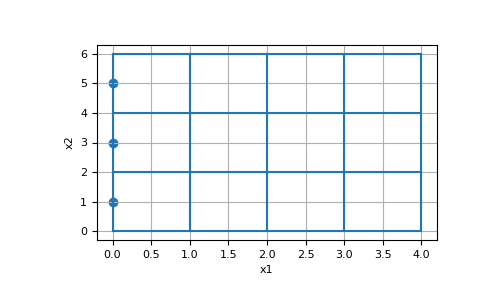# discretize.TensorMesh.face_boundary_indices¶

property TensorMesh.face_boundary_indices

Returns the indices of the x, (y and z) boundary faces

For x, (y and z) faces, this property returns the indices of the faces on the boundaries. That is, the property returns the indices of the x-faces that lie on the x-boundary; likewise for y and z. Note that each Cartesian direction will have both a lower and upper boundary, and the property will return the indices corresponding to the lower and upper boundaries separately.

E.g. for a 2D domain, there are 2 x-boundaries and 2 y-boundaries (4 in total). In this case, the return is a list of length 4 organized [ind_Bx1, ind_Bx2, ind_By1, ind_By2]:

```           By2
+ ------------- +
|               |
|               |
Bx1 |               | Bx2
|               |
|               |
+ ------------- +
By1
```
Returns
(`dim` * 2) `list` `of` `numpy.ndarray` `of` bool

The length of list returned depends on the dimension of the mesh. And the length of each array containing the indices depends on the number of faces in each direction. For 1D, 2D and 3D tensor meshes, the returns take the following form:

• 1D: returns [ind_Bx1, ind_Bx2]

• 2D: returns [ind_Bx1, ind_Bx2, ind_By1, ind_By2]

• 3D: returns [ind_Bx1, ind_Bx2, ind_By1, ind_By2, ind_Bz1, ind_Bz2]

Examples

Here, we construct a 4 by 3 cell 2D tensor mesh and return the indices of the x and y-boundary faces. In this case there are 3 x-faces on each x-boundary, and there are 4 y-faces on each y-boundary.

```>>> from discretize import TensorMesh
>>> import numpy as np
>>> import matplotlib.pyplot as plt
```
```>>> hx = [1, 1, 1, 1]
>>> hy = [2, 2, 2]
>>> mesh = TensorMesh([hx, hy])
>>> ind_Bx1, ind_Bx2, ind_By1, ind_By2 = mesh.face_boundary_indices
```
```>>> ax = plt.subplot(111)
>>> mesh.plot_grid(ax=ax)
>>> ax.scatter(*mesh.faces_x[ind_Bx1].T)
>>> plt.show()
```